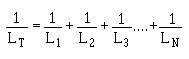mutual inductance is negligible. If there is no mutual inductance (magnetic coupling) and the inductors are connected in series, the total inductance is equal to the sum of the individual inductances. As a formula: ">Custom SearchSERIES INDUCTORS WITHOUT MAGNETIC COUPLING When inductors are well shielded or are located far enough apart from one another, the effect of mutual inductance is negligible. If there is no mutual inductance (magnetic coupling) and the inductors are connected in series, the total inductance is equal to the sum of the individual inductances. As a formula: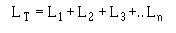where LT is the total inductance; L 1, L2, L3 are the inductances of L1, L2, L3; and Ln means that any number (n) of inductors may be used. The inductances of inductors in series are added together like the resistances of resistors in series. SERIES INDUCTORS WITH MAGNETIC COUPLING When two inductors in series are so arranged that the field of one links the other, the combined inductance is determined as follows: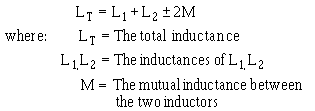The plus sign is used with M when the magnetic fields of the two inductors are aiding each other, as shown in figure 2-14.The minus sign is used with M when the magnetic field of the two inductors oppose each other, as shown in figure 2-15. The factor 2M accounts for the influence of L1 on L2 and L2 on L1. Figure 2-14. - Series inductors with aiding fields.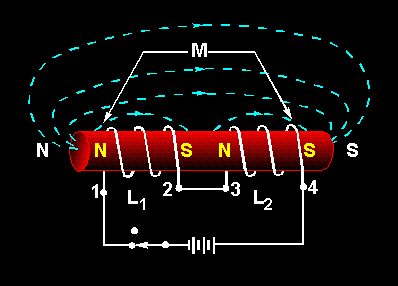Figure 2-15. - Series inductors with opposing fields.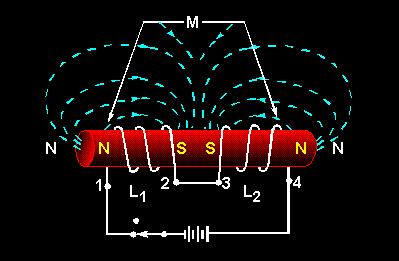A 10-H coil is connected in series with a 5-H coil so the fields aid each other. Their mutual inductance is 7 H. What is the combined inductance of the coils?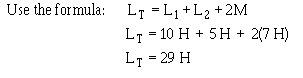Q.15 Two series-connected 7-H inductors are adjacent to each other; their coefficient of coupling is 0.64. What is the value of M? Q.16 A circuit contains two series inductors aligned in such a way that their magnetic fields oppose each other. What formula should you use to compute total inductance in this circuit? Q.17 The magnetic fields of two coils are aiding each other. The inductance of the coils are 3 H and 5 H, respectively. The coils' mutual inductance is 5 H. What is their combined inductance? PARALLEL INDUCTORS WITHOUT COUPLING The total inductance (LT) of inductors in parallel is calculated in the same manner that the total resistance of resistors in parallel is calculated, provided the coefficient of coupling between the coils is zero. Expressed mathematically: